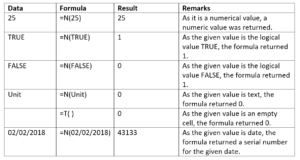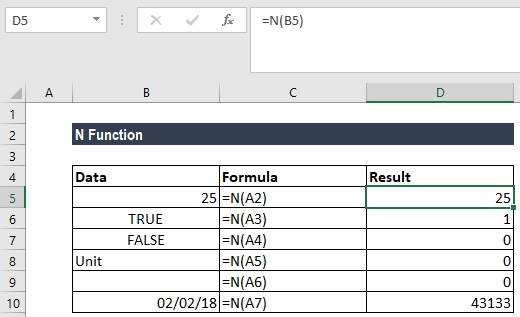# N Function

Returns a number for a value provided

## What is the N Function?

The N function is categorized under the Text functions in Excel. It will return a number when we provide a value. The function can be used to convert logical values TRUE and FALSE into 1 and 0, respectively.

As a financial analyst, the N function offers limited use, as MS Excel automatically converts values as necessary. The function is provided for compatibility with other spreadsheet programs.

### Formula

=N(value)

The formula uses only one argument:

1. Value (required argument) – This is the value that we wish to convert.

The N function will convert data into a numeric value, using the following rules:

Input valuesReturn value
Any numberSame number
A recognized dateA date is converted into Excel serial number format
TRUE1
FALSE0
An error code (#VALUE, #N/A, etc.)Same error code
Other values0

In Excel, dates are stored as sequential serial numbers so that we can use them in calculations. By default, January 1, 1900 is serial number 1, and January 1, 2018 is serial number 43101, as it is 43,101 days after January 1, 1900.

### How to use the N Function in Excel?

The N function is a built-in function in MS Excel. As a worksheet function, it can be entered into a formula in a worksheet cell.

To understand the uses of the function, let us consider an example:

#### Example 1

Suppose we are given the following data. Let us see how the function works:We get the results below:To show results for formulas in Excel, select the formulas, press F2, and then press Enter. If needed, you can increase the column width(s) to see all the results.

Thanks for reading CFI’s guide to the Excel N function. By taking the time to learn and master these functions, you’ll significantly speed up your financial modeling. To learn more, check out these additional CFI resources:

• Excel Functions for Finance